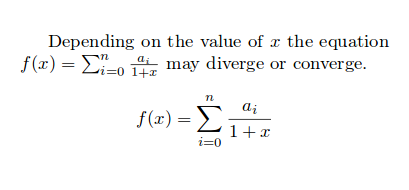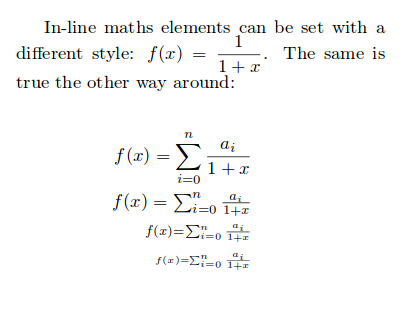Some mathematical elements change their style depending on the context, whether they are in line with the text or in an equation-type environment. This article explains how to manually adjust the display style.

# Introduction

Let's see an example

Depending on the value of $x$ the equation $$f(x) = \sum_{i=0}^{n} \frac{a_i}{1+x}$$ may diverge or converge.

$f(x) = \sum_{i=0}^{n} \frac{a_i}{1+x}$Superscripts, subscripts and fractions are formatted differently.

# Setting mathematical styles

The maths styles can be set explicitly. For instance, if you want an in-line mathematical element to display as a equation-like element put \displaystyle before that element. There are some more maths style-related commands that change the size of the text.

In-line maths elements can be set with a different style: $$f(x) = \displaystyle \frac{1}{1+x}$$. The same is true the other way around:

\begin{eqnarray*}
\begin{eqnarray*}
f(x) = \sum_{i=0}^{n} \frac{a_i}{1+x} \\
\textstyle f(x) = \textstyle \sum_{i=0}^{n} \frac{a_i}{1+x} \\
\scriptstyle f(x) = \scriptstyle \sum_{i=0}^{n} \frac{a_i}{1+x} \\
\scriptscriptstyle f(x) = \scriptscriptstyle \sum_{i=0}^{n} \frac{a_i}{1+x}
\end{eqnarray*}
\end{eqnarray*}